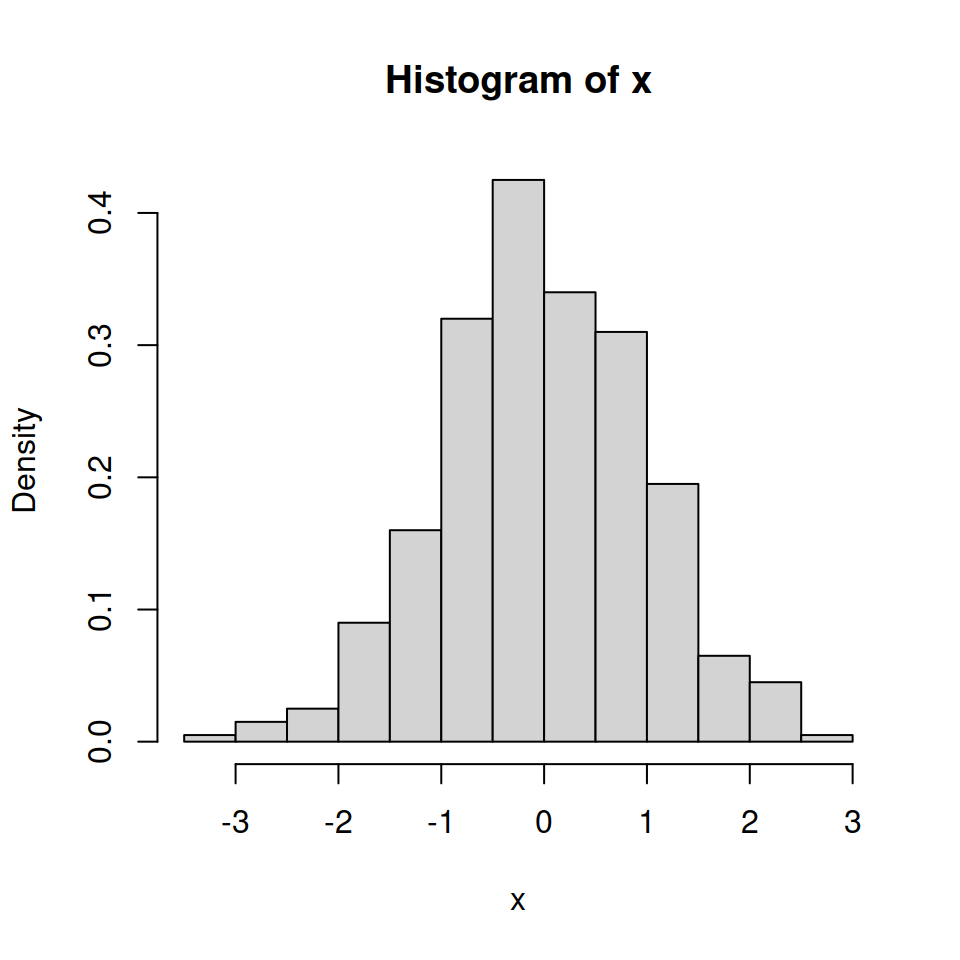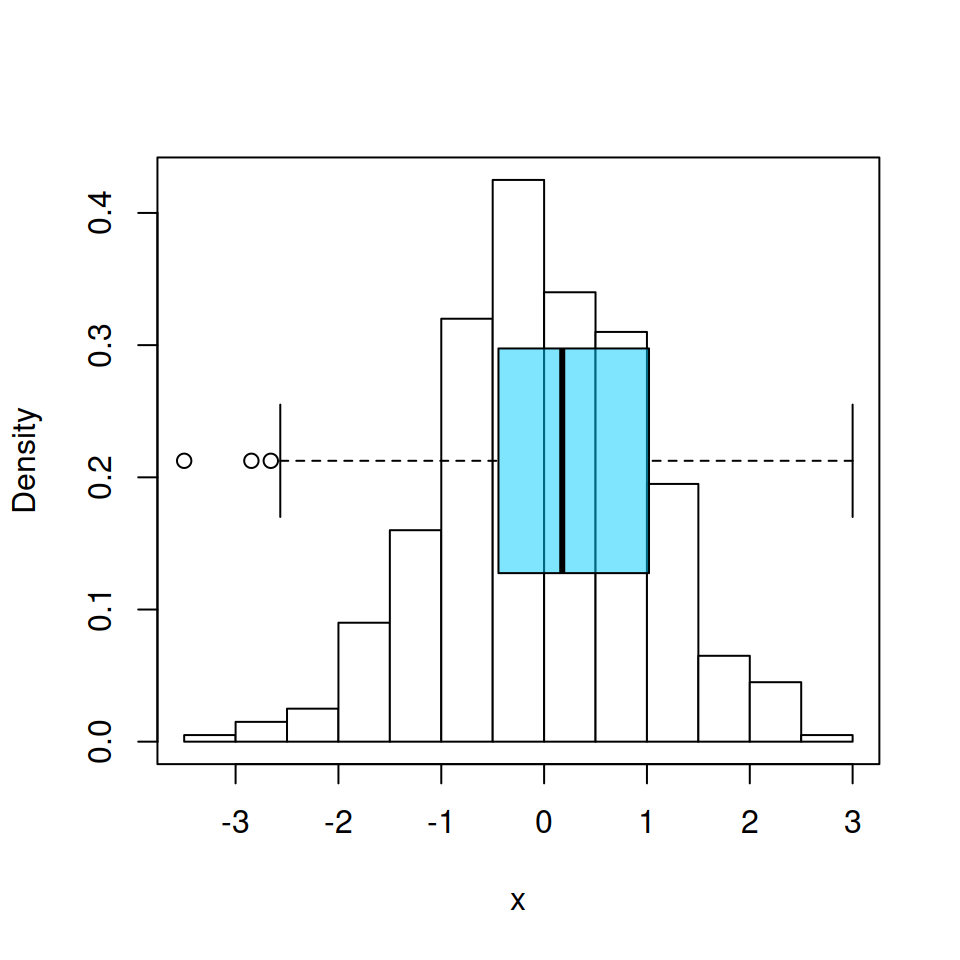# Histogram and boxplot in R

A histogram can be created in R with the `hist` function. By default a frequency histogram will be created, but you can create a density histogram setting `prob = TRUE`. A box plot can be created with the `boxplot` function.

``````# Sample data
set.seed(5)
x <- rnorm(400)

# Histogram
hist(x, prob = TRUE)``````## Histogram and boxplot

Sometimes it is interesting to overlay a box plot over a histogram. For that purpose you will need to use `par(new = TRUE)` to add a plot over the previous, removing the axes of the box plot with `axes = FALSE`, setting it horizontal with `horizontal = TRUE` and using a color with transparency making use of `rgb` to avoid hiding the histogram.Box plot over an histogram

``````# Histogram
hist(x, prob = TRUE,
col = "white",
main = "")